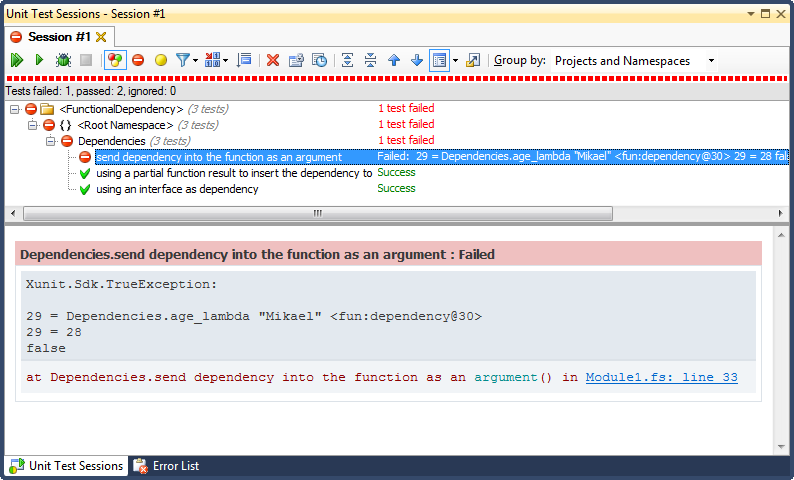# Dealing with dependencies in functional programming

What is a dependency?

• Some entity that your unit depends on. (too abstract description)
• Something that you want to replace while unit testing (too concrete description)

In OO programming you will use a dependency injection framework to build dependency chains. You use it to inject dependencies into constructors, methods and parameters. That is all very cool and enables testability, but not very useful in functional programming.

### Why do we need to think about dependencies in functional programming?

• Testing your units are still important, and to enable that you need to stub away your dependencies
• Coupling also exists in functional programming and should be considered an evil

## Function argument dependency injection

The most obvious way to handle dependencies is to send them into the function as an argument.

``````// Model object
type Person = { Name : string; BirthDate : System.DateTime }

// age: get the age of a person from name
// string -> (string -> Person) -> int
let age name (fn_getPerson : string -> Person) =

// Get birthdate from dependency
let birthDate = (fn_getPerson name).BirthDate

// Calculate
(DateTime.Now - birthDate).Days / 365
``````
``````val age : string -> (string -> Person) -> int
``````

The dependency is a function that knows how to get a person from the database by calling with its name. While unit testing we really want to get rid of that dependency and that is why we send it in as a function, instead of calling it directly from where we need its result.

```age "Mikael Lundin" DataAccess.GetPerson
// or maybe
DataAccess.GetPerson |> age "Mikael Lundin"```

Testing would look like this. Here I'm using xUnit and Unquote for testing.

``````open Xunit
open Swensen.Unquote

[<Fact>]
let ``send dependency into the function as an argument`` () =
// Setup: In our test we return a Person directly
let dependency (s : string) = { Name = s; BirthDate = new DateTime(1982, 7, 15) }

// Assert
test <@ 29 = age "Mikael" dependency  @>
``````

Unit testing with Xunit and Unquote is a blast. I love the expressiveness that Unquote gives us. If we where to run this with Resharper as testrunner, and fail the test - we get this great test failure.Oh, I love it!

### Partial function application injection

This is a very scary title, but another functional way to deal with dependencies. Instead of accepting the dependency as an argument, you return a function that have to be completed with the dependency to run.

``````// Dependency through partial function
let age name : ((string -> Person) -> int) =
// Return a function
(fun (getPerson : string -> Person) ->
// Get birthdate
let birthDate = getPerson(name).BirthDate

// Return age
(DateTime.Now - birthDate).Days / 365
)
``````
``````val age : string -> (string -> Person) -> int
``````

This is indeed a bit harder to read and makes the function signature a bit messy. I return a function that will accept the dependency as an argument. Anyway I do like this method better than the previous.

```age "Mikael" DataAccess.GetPerson
// this also works
DataAccess.GetPerson |> age "Mikael"```

And here's a test.

``````[<Fact>]
let ``using a partial function result to insert the dependency to`` () =
// Setup: Our dependency is called on the function result
let dependency s = { Name = s; BirthDate = new DateTime(1982, 7, 15) }

// Assert
test <@ 28 = (age "Mikael") dependency @>
``````

### Interface dependency injection

Since F# has object literals, it is very easy to stub out interfaces, and when talking to OO libraries you might have to resort to this method. Consider our dependency.

```public interface IUserRepository
{
IEnumerable<User> GetUsers();
}

public class User
{
public string UserName { get; set; }
```public string Password { get; set; }
```
}```

We would like to write an authenticate method in F#. How should we handle our dependency? Here's some F# equivalient of what we would do in OOP.

```let authenticate username password (rep : IUserRepository) =

When calling this from a test, we can use object literals to change the implementation of the interface members.

``````[<Fact>]
let ``using an interface as dependency`` () =
// GetUsers() result
let users = [| new User(UserName = "mlu", Password = "secret") |]

// Fake repository
let repository = {
new IUserRepository with
member x.GetUsers() = users |> Seq.ofArray
}

// Assert
test <@ true = authenticate "mlu" "secret" repository @>
``````

But a more functional approach would be, not to use the interface as a dependency, but focus on the actual function as we did on the first two patterns. Let the caller of this function worry about on what object instance the dependent function lives.

```let authenticate username password : ((unit -> seq<User>) -> bool) =
(fun (getUsers : unit -> seq<User>) ->
getUsers() |> Seq.exists
``````val authenticate : string -> string -> (unit -> seq<User>) -> bool